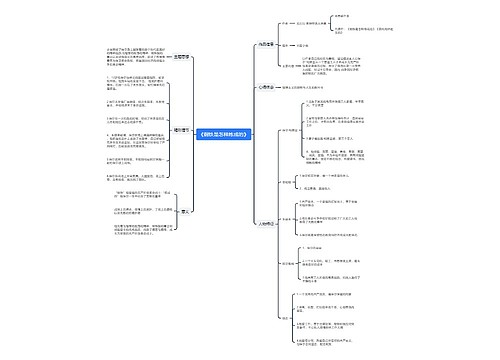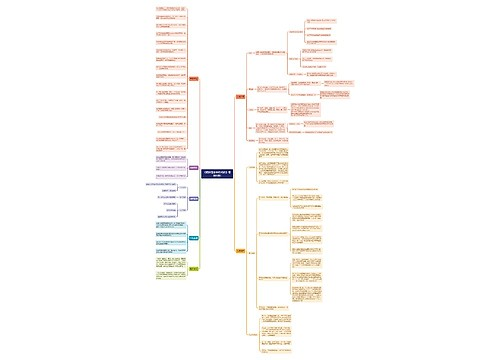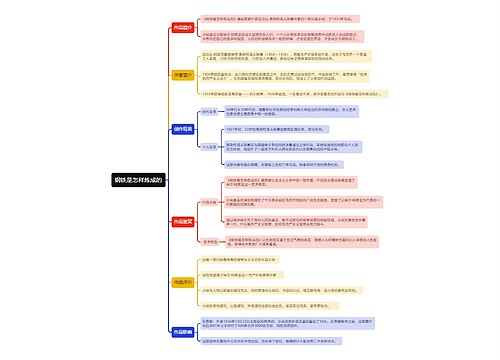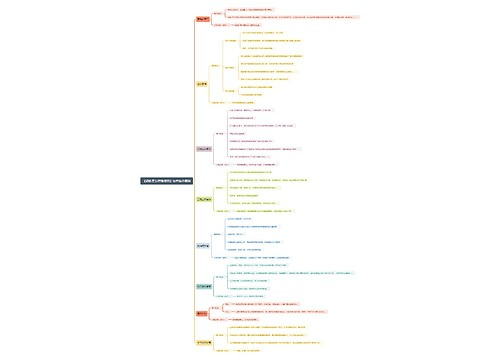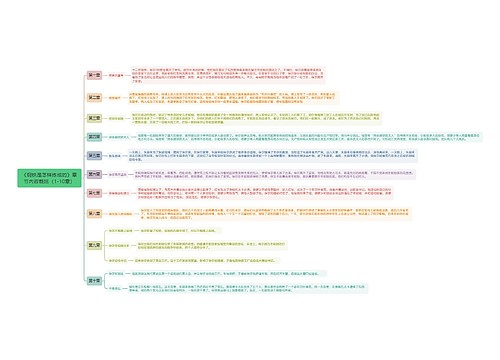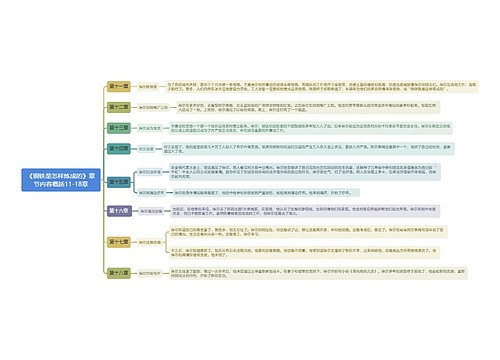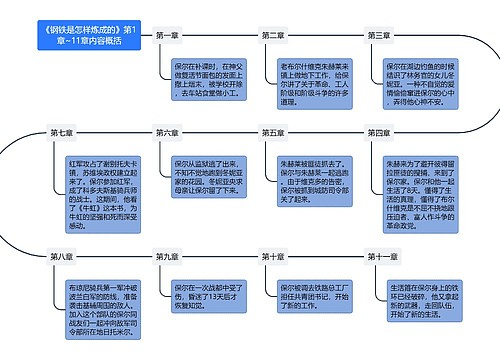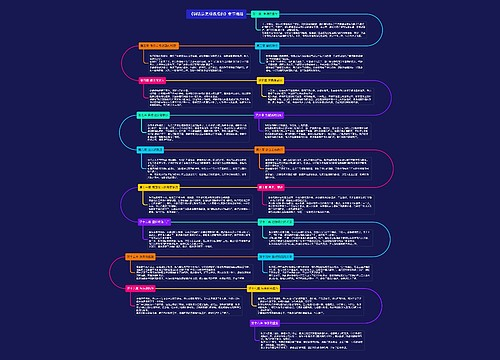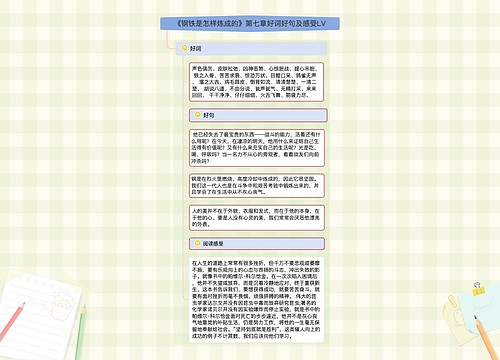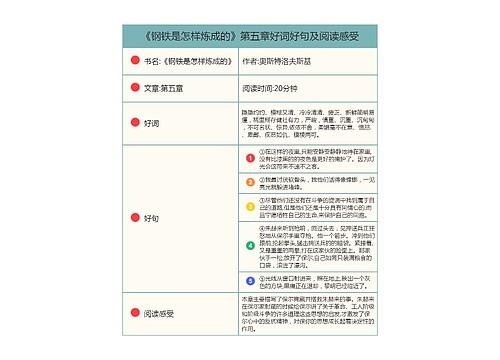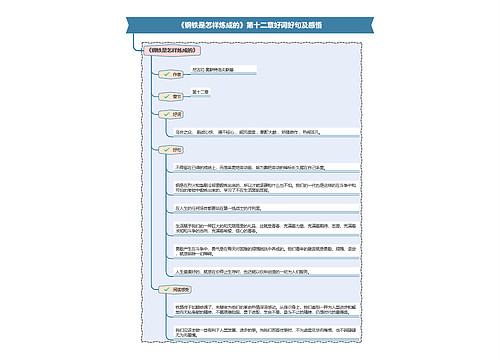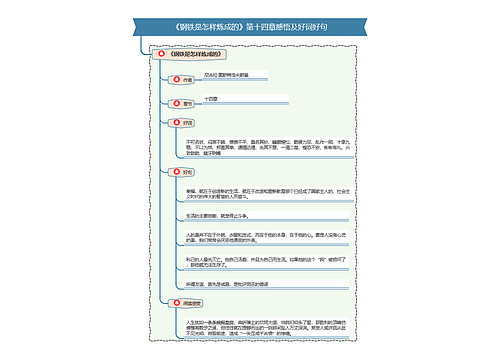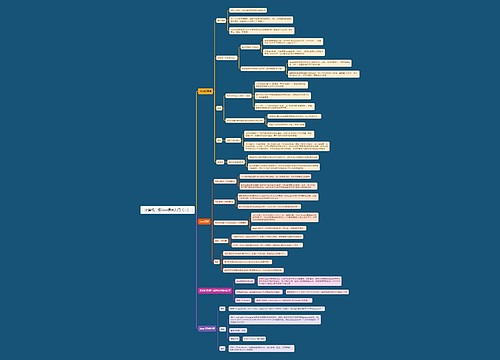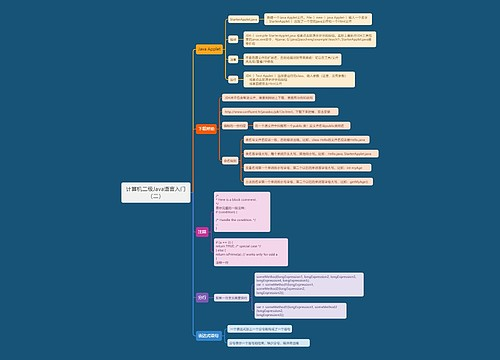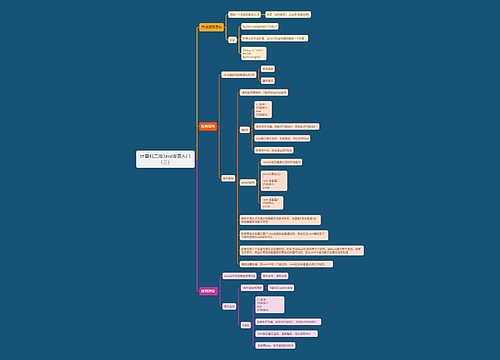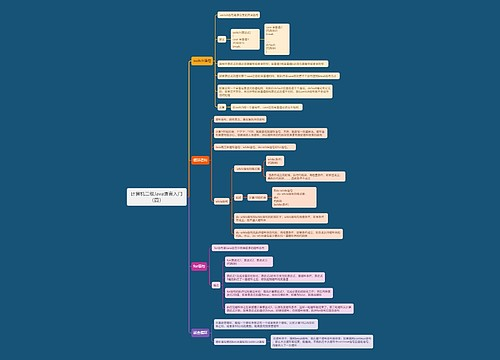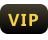成为

# 求n以内的素数-从入门到入坟思维导图

收藏
分享U125568174  浏览量：1532023-02-09 20:24:33

## 思维导图大纲

• 求n以内的素数 -从入门到入坟思维导图模板大纲

1. ## 1、试除法

1. ﻿最简单的方法，对n以内所有数进行试除

2. 要点

1. ﻿1、开始前判断是否大于1，不满足直接退出，因为0，1不是素数

2. ﻿2、for循环内条件写i*i<=n，减少不必要的判断，比sqrt()省时间

3. bool prime(int x){ if(x<=1) return 0; for(int i=2;i*i<=n;i++){ if(x%i==0) return 0; } return 1; }

4. ﻿缺点

1. ﻿每一个素数都要进行较长的计算，费时间

2. ﻿由于n以内素数约为n/ln(n)个，所以时间复杂度为O(n*sqrt(n)/ln(n)), 较为费时间

2. ## 2、朴素筛

1. 原理

1. ﻿将所有数的n倍(n≥2)全部删除，因为他们一定不是素数

2. for(int i=2;i<=n;i++){ for(int j=2;i*j<=n;j++){ book[i*j]=1; } }

3. ﻿时间复杂度略有降低，约n*log2(n)

4. ﻿缺点

1. ﻿需要对每一个数的倍数筛除，即使是合数的倍数，极其浪费时间

2. ﻿例如12=2*6=3*4，则它被筛了4次

3. ## 3、埃氏筛

1. ﻿在朴素筛的基础上加入一条判断条件以及第二重循环小优化

2. for(int i=2;i<=n;i++){ if(book[i]==0){ for(int j=i;i*j<=n;j++){ book[i*j]=1; } } }

3. ﻿时间复杂度大大降低，约为O(NlnlnN)，已经接近于O(N)级别

4. ﻿缺点

1. ﻿例如12=2*4=2*6，则12总计被筛了两次，以此类推

4. ## ﻿4、欧拉筛（线性筛）

1. ﻿由于埃氏筛任由重复运算，其实并不是最快

2. ﻿欧拉筛将素数存储在数组中，第二重循环将i的prime[j]倍的数筛除，同时当i是prime[j]的倍数是退出循环。保证每个合数只计算一次

3. for(int i=2;i<=n-1;i++){ if(book[i]==0){ cnt++; prime[cnt]=i; } for(int j=1;prime[j]*i<n;j++){ book[i*prime[j]]=1; if(i%prime[j]==0) break; } }

4. ﻿因其对每一个合数只会筛除一次，时间复杂度为O(n)，目前最快的筛法

## 相关思维导图模板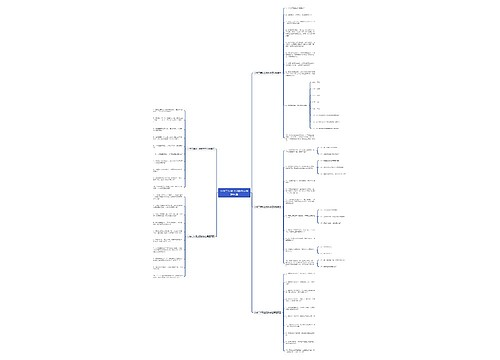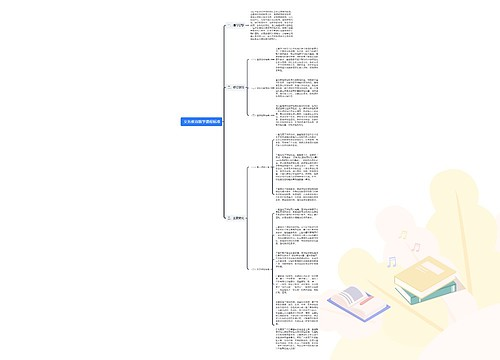### 相关思维导图专辑